The Australian Journal of Mathematical Analysis and Applications

 Home News Editors Volumes RGMIA Subscriptions Authors Contact

ISSN 1449-5910

Sorry death is imminent for file: ../public_html/searchroot/files/tex/v19n2/v19i2p4.tex
You searched for park
Total of 103 results found in site

16: Paper Source PDF document

Paper's Title:

Fixed Points and Stability of the Cauchy Functional Equation

Author(s):

Choonkil Park and Themistocles M. Rassias

Department of Mathematics, Hanyang University,
Seoul 133-791,
Republic of Korea

Department of Mathematics, National Technical University of Athens,
Zografou Campus, 15780 Athens,
Greece

baak@hanyang.ac.kr
trassias@math.ntua.gr

Abstract:

Using fixed point methods, we prove the generalized Hyers-Ulam stability of homomorphisms in Banach algebras and of derivations on Banach algebras for the Cauchy functional equation.

13: Paper Source PDF document

Paper's Title:

Approximation of an AQCQ-Functional Equation and its Applications

Author(s):

Choonkil Park and Jung Rye Lee

Department of Mathematics,
Research Institute for Natural Sciences,
Hanyang University, Seoul 133-791,
Korea;

Department of Mathematics,
Daejin University,
Kyeonggi 487-711,
Korea

baak@hanyang.ac.kr
jrlee@daejin.ac.kr

Abstract:

This paper is a survey on the generalized Hyers-Ulam stability of an AQCQ-functional equation in several spaces. Its content is divided into the following sections:

1. Introduction and preliminaries.

2. Generalized Hyers-Ulam stability of an AQCQ-functional equation in Banach spaces: direct method.

3. Generalized Hyers-Ulam stability of an AQCQ-functional equation in Banach spaces: fixed point method.

4. Generalized Hyers-Ulam stability of an AQCQ-functional equation in random Banach spaces: direct method.

5. Generalized Hyers-Ulam stability of an AQCQ-functional equation in random Banach spaces: fixed point method.

6. Generalized Hyers-Ulam stability of an AQCQ-functional equation in non-Archi-medean Banach spaces: direct method.

7. Generalized Hyers-Ulam stability of an AQCQ-functional equation in non-Archi-medean Banach spaces: fixed point method.

13: Paper Source PDF document

Paper's Title:

Ostrowski Type Inequalities for Lebesgue Integral: a Survey of Recent Results

Author(s):

Sever S. Dragomir1,2

1Mathematics, School of Engineering & Science
Victoria University, PO Box 14428
Melbourne City, MC 8001,
Australia
E-mail: sever.dragomir@vu.edu.au

2DST-NRF Centre of Excellence in the Mathematical and Statistical Sciences,
School of Computer Science & Applied Mathematics,
University of the Witwatersrand,
Private Bag 3, Johannesburg 2050,
South Africa
URL: http://rgmia.org/dragomir

Abstract:

The main aim of this survey is to present recent results concerning Ostrowski type inequalities for the Lebesgue integral of various classes of complex and real-valued functions. The survey is intended for use by both researchers in various fields of Classical and Modern Analysis and Mathematical Inequalities and their Applications, domains which have grown exponentially in the last decade, as well as by postgraduate students and scientists applying inequalities in their specific areas.

6: Paper Source PDF document

Paper's Title:

Linearly Transformable Minimal Surfaces

Author(s):

Harold R. Parks and Walter B. Woods

Department of Mathematics, Oregon State University,
Corvallis, Oregon 97331--4605,
USA
parks@math.oregonstate.edu
URL
: http://www.math.oregonstate.edu/people/view/parks/

Abstract:

We give a complete description of a nonplanar minimal surface in R3 with the surprising property that the surface remains minimal after mapping by a linear transformation that dilates by three distinct factors in three orthogonal directions. The surface is defined in closed form using Jacobi elliptic functions.

6: Paper Source PDF document

Paper's Title:

Purely Unrectifiable Sets with Large Projections

Author(s):

Harold R. Parks

Department of Mathematics, Oregon State University,
Corvallis, Oregon 97331--4605,
USA
parks@math.oregonstate.edu
URL
: http://www.math.oregonstate.edu/people/view/parks/

Abstract:

For n2, we give a construction of a compact subset of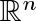that is dispersed enough that it is purely unrectifiable, but that nonetheless has an orthogonal projection that hits every point of an (n-1)-dimensional unit cube. Moreover, this subset has the additional surprising property that the orthogonal projection onto any straight line inis a set of positive 1-dimensional Hausdorff measure.

5: Paper Source PDF document

Paper's Title:

Applications of Relations and Relators in the Extensions of Stability Theorems for Homogeneous and Additive Functions

Author(s):

Árpád Száz

Institute of Mathematics, University of Debrecen,
H-4010 Debrecen, Pf. 12,
Hungary
szaz@math.klte.hu

Abstract:

By working out an appropriate technique of relations and relators and extending the ideas of the direct methods of Z. Gajda and R. Ger, we prove some generalizations of the stability theorems of D. H. Hyers, T. Aoki, Th. M. Rassias and P. Găvruţă in terms of the existence and unicity of 2-homogeneous and additive approximate selections of generalized subadditive relations of semigroups to vector relator spaces. Thus, we obtain generalizations not only of the selection theorems of Z. Gajda and R. Ger, but also those of the present author.

4: Paper Source PDF document

Paper's Title:

Hyers-Ulam-Rassias Stability of a Generalized Jensen Functional Equation

Author(s):

A. Charifi, B. Bouikhalene, E. Elqorachi and A. Redouani

Department of
Mathematics, Faculty of Sciences,
Ibn Tofail University,
Kenitra, Morocco
charifi2000@yahoo.fr bbouikhalene@yahoo.fr

Department of
Mathematics, Faculty of Sciences,
Ibn Zohr University,
elqorachi@hotmail.com Redouani-ahmed@yahoo.fr

Abstract:

In this paper we obtain the Hyers-Ulam-Rassias stability for the generalized Jensen's functional equation in abelian group (G,+). Furthermore we discuss the case where G is amenable and we give a note on the Hyers-Ulam-stability of the K-spherical (n × n)-matrix functional equation.

4: Paper Source PDF document

Paper's Title:

Ulam Stability of Reciprocal Difference and Adjoint Functional Equations

Author(s):

K. Ravi, J. M. Rassias and B. V. Senthil Kumar

Department of Mathematics,
Sacred Heart College, Tirupattur - 635601,
India

Pedagogical Department E. E.,
Section of Mathematics and Informatics,
National and Capodistrian University of Athens,
Athens, Attikis 15342,
GREECE

Department of Mathematics,
C.Abdul Hakeem College of Engineering and
Technology, Melvisharam - 632 509, India

shckavi@yahoo.co.in
jrassias@primedu.uoa.gr
bvssree@yahoo.co.in

Abstract:

In this paper, the reciprocal difference functional equation (or RDF equation) and the reciprocal adjoint functional equation (or RAF equation) are introduced. Then the pertinent Ulam stability problem for these functional equations is solved, together with the extended Ulam (or Rassias) stability problem and the generalized Ulam (or Ulam-Gavruta-Rassias) stability problem for the same equations.

4: Paper Source PDF document

Paper's Title:

On the Hyers-Ulam Stability of Homomorphisms and Lie Derivations

Author(s):

Department of Mathematics, Payame Noor University,
P.O. Box: 19395-3697, Tehran,
Iran.

Abstract:

Let A be a Lie Banach*-algebra. For each elements (a, b) and (c, d) in A2:= A * A, by definitions

(a, b) (c, d)= (ac, bd),
|(a, b)|= |a|+ |b|,
(a, b)*= (a*, b*),

A2 can be considered as a Banach*-algebra. This Banach*-algebra is called a Lie Banach*-algebra whenever it is equipped with the following definitions of Lie product: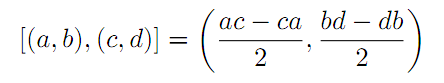for all a, b, c, d in A. Also, if A is a Lie Banach*-algebra, then D: A2→A2 satisfying

D ([ (a, b), (c, d)])= [ D (a, b), (c, d)]+ [(a, b), D (c, d)]

for all \$a, b, c, d∈A, is a Lie derivation on A2. Furthermore, if A is a Lie Banach*-algebra, then D is called a Lie* derivation on A2 whenever D is a Lie derivation with D (a, b)*= D (a*, b*) for all a, b∈A. In this paper, we investigate the Hyers-Ulam stability of Lie Banach*-algebra homomorphisms and Lie* derivations on the Banach*-algebra A2.

3: Paper Source PDF document

Paper's Title:

Stability of a Mixed Additive, Quadratic and Cubic Functional Equation In Quasi-Banach Spaces

Author(s):

Department of Mathematics, Faculty of Sciences,
University of Mohaghegh Ardabili, Ardabil,
Iran
a.nejati@yahoo.com

Faculty of Mathematical Sciences,
University of Tabriz, Tabriz,
Iran

Abstract:

In this paper we establish the general solution of a mixed additive, quadratic and cubic functional equation and investigate the Hyers--Ulam--Rassias stability of this equation in quasi-Banach spaces. The concept of Hyers-Ulam-Rassias stability originated from Th. M. Rassias' stability theorem that appeared in his paper: On the stability of the linear mapping in Banach spaces, Proc. Amer. Math. Soc. 72 (1978), 297--300.

3: Paper Source PDF document

Paper's Title:

Some properties of quasinormal, paranormal and 2-k* paranormal operators

Author(s):

Shqipe Lohaj

Department of Mathematics,
University of Prishtina,
10000, Kosova.
E-mail: shqipe.lohaj@uni-pr.edu

Abstract:

In the beginning of this paper some conditions under which an operator is partial isometry are given. Further, the class of 2-k* paranormal operators is defined and some properties of this class in Hilbert space are shown. It has been proved that an unitarily operator equivalent with an operator of a 2-k* paranormal operator is a 2-k* paranormal operator, and if is a 2-k* paranormal operator, that commutes with an isometric operator, then their product also is a \$2-k^*\$ paranormal operator.

2: Paper Source PDF document

Paper's Title:

A general common fixed point theorem for reciprocally continuous mappings satisfying an implicit relation

Author(s):

A. Djoudi and A. Aliouche

Faculty of Science, University of Annaba,
P.O. Box 23000, Annaba,
Algeria.

Department of Mathematics, University of Larbi Ben M'Hidi,
Oum-El-Bouaghi 04000,
Algeria.
abdmath@hotmail.com

Abstract:

A general common fixed point theorem for compatible mappings satisfying an implicit relation is obtained by replacing the continuity of one mapping by the reciprocal continuity of two mappings.

2: Paper Source PDF document

Paper's Title:

The Convergence of Modified Mann-Ishikawa Iterations when Applied to an Asymptotically Pseudocontractive Map

Author(s):

S. Soltuz

Departamento de Matematicas, Universidad de Los Andes, Carrera 1
No. 18A-10, Bogota,
Colombia
and
``T. Popoviciu" Institute of Numerical Analysis
Cluj-Napoca,
Romania
smsoltuz@gmail.com
URL:http://www.uniandes.edu.co/

Abstract:

We prove that under minimal conditions the modified Mann and Ishikawa iterations converge when dealing with an asymptotically pseudocontractive map. We give an affirmative answer to the open question from C.E. Chidume and H. Zegeye, Approximate fixed point sequences and convergence theorems for asymptotically pseudocontractive mappings, J. Math. Anal. Appl., 278 (2003), 354--366.

2: Paper Source PDF document

Paper's Title:

On Stan Ulam and his Mathematics

Author(s):

Krzysztof Ciesielski and Themistocles M. Rassias

Mathematics Institute, Jagiellonian University,
Ł
jasiewicza 6, 30-348 Kraków,
Poland
Department of Mathematics. National Technical University of Athens,
Zografou Campus, 15780 Athens,
Greece

Krzysztof.Ciesielski@im.uj.edu.pl
trassias@math.ntua.gr

Abstract:

In this note we give a glimpse of the curriculum vitae of Stan Ulam, his personality and some of the mathematics he was involved in.

2: Paper Source PDF document

Paper's Title:

Ostrowski Type Fractional Integral Inequalities for Generalized (s,m,φ)-preinvex Functions

Author(s):

Artion Kashuri and Rozana Liko

University of Vlora "Ismail Qemali",
Faculty of Technical Science,
Department of Mathematics, 9400,
Albania.
E-mail: artionkashuri@gmail.com
E-mail: rozanaliko86@gmail.com

Abstract:

In the present paper, the notion of generalized (s,m,φ)-preinvex function is introduced and some new integral inequalities for the left hand side of Gauss-Jacobi type quadrature formula involving generalized (s,m,φ)-preinvex functions along with beta function are given. Moreover, some generalizations of Ostrowski type inequalities for generalized (s,m,φ)-preinvex functions via Riemann-Liouville fractional integrals are established.

2: Paper Source PDF document

Paper's Title:

A Caratheodory's Approximate Solutions of Stochastic Differential Equations Under the Hölder Condition

Author(s):

Bo-Kyeong Kim and Young-Ho Kim

Department of Mathematics,
Changwon National University,
Changwon, Gyeongsangnam-do 51140,
Korea.
E-mail: claire9576@naver.com
yhkim@changwon.ac.kr

Abstract:

In this paper, based on the theorem of the uniqueness of the solution of the stochastic differential equation, the convergence possibility of the Caratheodory's approximate solution was studied by approximating the unique solution. To obtain this convergence theorem, we used a Hölder condition and a weakened linear growth condition. Furthermore, The auxiliary theorems for the existence and continuity of the Caratheodory's approximate solution were investigated as a prerequisite.

2: Paper Source PDF document

Paper's Title:

Numerical Study of a Mathematical Model of a Free-Surface Potential Flow

Author(s):

H. Serguine, F. Guechi and A. Gasmi

Department Of Mathematics, Faculty of Science,
Ferhat Abbas Universty, 19000, Setif,
Algeria.
E-mail: houria.serguine@univ-msila.dz

Department Of Mathematics, Faculty of Science,
Ferhat Abbas Universty, 19000, Setif,
Algeria.
E-mail:  fairouz.chegaar@univ-setif.dz

Laboratory of Pure and Applied Mathematics, Faculty of Mathematics and Computer Science,
Mohamed Boudiaf Universty, 28000, M'sila,
Algeria.

Abstract:

In this work, the problem of a potential and two-dimensional flow with a free surface of an incompressible, irrotational and inviscid fluid of a jet in front an inclined wall is considered, where γ is the inclination angle with the horizontal. The shape of the free surface is presented by curves which are found numerically by the series truncation method. This technique is based on the conformal transformations, resulting with the surface tension effect T with the boundary conditions on the free surfaces given by Bernoulli's equation. The found results are dependant on parameters which are: the Weber's number α and the angle γ. For each Weber's number value, only one solution is specified and some shapes of free surfaces of the jet are illustrated.

1: Paper Source PDF document

Paper's Title:

On a Generalized Biharmonic Equation in Plane Polars with Applications to Functionally Graded Materials

Author(s):

Ciro D'Apice

Department of Information Engineering and Applied Mathematics (DIIMA),
University of Salerno, 84084 Fisciano (SA),
Salerno, Italy.
dapice@diima.unisa.it

Abstract:

In this paper we consider a generalized biharmonic equation modelling a two-dimensional inhomogeneous elastic state in the curvilinear rectangle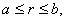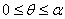where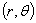denote plane polar coordinates. Such an arch--like region is maintained in equilibrium under self--equilibrated traction applied on the edge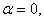while the other three edges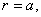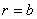and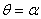are traction free. Our aim is to derive some explicit spatial exponential decay bounds for the specific Airy stress function and its derivatives. Two types of smoothly varying inhomogeneity are considered: (i) the elastic moduli vary smoothly with the polar angle, (ii) they vary smoothly with the polar distance. Such types of smoothly varying inhomogeneous elastic materials provide a model for technological important functionally graded materials. The results of the present paper prove how the spatial decay rate varies with the constitutive profile.

1: Paper Source PDF document

Paper's Title:

Reconstruction of Discontinuities of Functions Given Noisy Data

Author(s):

Eric D. Mbakop

67A Beaver Park Rd,
Framingham, MA, 01702,
U. S. A.
ericsteve86@yahoo.fr

Abstract:

Suppose one is given noisy data of a discontinuous piecewise-smooth function along with a bound on its second derivative. The locations of the points of discontinuity of f and their jump sizes are not assumed known, but are instead retrieved stably from the noisy data. The novelty of this paper is a numerical method that allows one to locate some of these points of discontinuity with an accuracy that can be made arbitrarily small.

1: Paper Source PDF document

Paper's Title:

On the Generalized Stability and Asymptotic Behavior of Quadratic Mappings

Author(s):

Hark-Mahn Kim, Sang-Baek Lee and Eunyoung Son

Department of Mathematics
Chungnam National University
Daejeon, 305-764,
Republic of Korea

hmkim@cnu.ac.kr

Abstract:

We extend the stability of quadratic mappings to the stability of general quadratic mappings with several variables, and then obtain an improved asymptotic property of quadratic mappings on restricted domains.

1: Paper Source PDF document

Paper's Title:

Stability of Almost Multiplicative Functionals

Author(s):

Norio Niwa, Hirokazu Oka, Takeshi Miura and Sin-Ei Takahasi

Faculty of Engineering, Osaka Electro-Communication University,
Neyagawa 572-8530,
Japan

Faculty of Engineering, Ibaraki University,
Hitachi 316-8511,
Japan

Department of Applied Mathematics and Physics, Graduate School of Science and Engineering,
Yamagata University,
Yonezawa 992-8510
Japan

oka@mx.ibaraki.ac.jp
miura@yz.yamagata-u.ac.jp
sin-ei@emperor.yz.yamagata-u.ac.jp

Abstract:

Let δ and p be non-negative real numbers. Let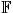be the real or complex number field and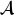a normed algebra over. If a mapping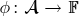satisfies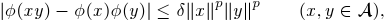then we show that φ is multiplicative or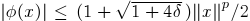for all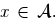If, in addition, φ satisfies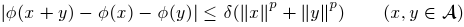for some p1, then by using Hyers-Ulam-Rassias stability of additive Cauchy equation, we show that φ is a ring homomorphism or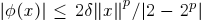for all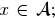In other words, φ is a ring homomorphism, or an approximately zero mapping. The results of this paper are inspired by Th.M. Rassias' stability theorem.

1: Paper Source PDF document

Paper's Title:

A Coincidence Theorem for Two Kakutani Maps

Author(s):

Mircea Balaj

Department of Mathematics,
Romania.

Abstract:

In this paper we prove the following theorem: Let X be a nonempty compact convex set in a locally convex Hausdorff topological vector space, D be the set of its extremal points and F,T: XX two Kakutani maps; if for each nonempty finite subset A of D and for any x ∈ coA, F (x) coA ≠ Ř, then F and T have a coincidence point. The proof of this theorem is given first in the case when X is a simplex, then when X is a polytope and finally in the general case. Several reformulations of this result are given in the last part of the paper.

1: Paper Source PDF document

Paper's Title:

Some New Generalizations of Jensen's Inequality with Related Results and Applications

Author(s):

Steven G. From

Department of Mathematics

E-mail: sfrom@unomaha.edu

Abstract:

In this paper, some new generalizations of Jensen's inequality are presented. In particular, upper and lower bounds for the Jensen gap are given and compared analytically and numerically to previously published bounds for both the discrete and continuous Jensen's inequality cases. The new bounds compare favorably to previously proposed bounds. A new method based on a series of locally linear interpolations is given and is the basis for most of the bounds given in this paper. The wide applicability of this method will be demonstrated. As by-products of this method, we shall obtain some new Hermite-Hadamard inequalities for functions which are 3-convex or 3-concave. The new method works to obtain bounds for the Jensen gap for non-convex functions as well, provided one or two derivatives of the nonlinear function are continuous. The mean residual life function of applied probability and reliability theory plays a prominent role in construction of bounds for the Jensen gap. We also present an exact integral representation for the Jensen gap in the continuous case. We briefly discuss some inequalities for other types of convexity, such as convexity in the geometric mean, and briefly discuss applications to reliability theory.

1: Paper Source PDF document

Paper's Title:

A Note on Divergent Fourier Series and λ-Permutations

Author(s):

A. Castillo, J. Chavez and H. Kim

Tufts University,
Department of Mathematics,
Medford, MA 02155,
USA
E-mail: angel.castillo@tufts.edu

Texas Tech University,
Department of Mathematics and Statistics,
Lubbock, TX 79409,
USA
E-mail: josechavez5@my.unt.edu

University of Michigan-Dearborn,
Department of Mathematics and Statistics,
Dearborn, MI 48128,
USA.
E-mail: khyejin@umich.edu

Abstract:

We present a continuous function on [-π,π] whose Fourier series diverges and it cannot be rearranged to converge by a λ-permutation.

1: Paper Source PDF document

Paper's Title:

Inequalities for Discrete F-Divergence Measures: A Survey of Recent Results

Author(s):

Sever S. Dragomir1,2

1Mathematics, School of Engineering & Science
Victoria University, PO Box 14428
Melbourne City, MC 8001,
Australia
E-mail: sever.dragomir@vu.edu.au

2DST-NRF Centre of Excellence in the Mathematical and Statistical Sciences,
School of Computer Science & Applied Mathematics,
University of the Witwatersrand,
Private Bag 3, Johannesburg 2050,
South Africa
URL: http://rgmia.org/dragomir

Abstract:

In this paper we survey some recent results obtained by the author in providing various bounds for the celebrated f-divergence measure for various classes of functions f. Several techniques including inequalities of Jensen and Slater types for convex functions are employed. Bounds in terms of Kullback-Leibler Distance, Hellinger Discrimination and Varation distance are provided. Approximations of the f-divergence measure by the use of the celebrated Ostrowski and Trapezoid inequalities are obtained. More accurate approximation formulae that make use of Taylor's expansion with integral remainder are also surveyed. A comprehensive list of recent papers by several authors related this important concept in information theory is also included as an appendix to the main text.

1: Paper Source PDF document

Paper's Title:

A Low Order Least-Squares Nonconforming Finite Element Method for Steady Magnetohydrodynamic Equations

Author(s):

Z. Yu, D. Shi and H. Zhu

College of Science,
Zhongyuan University of Technology,
Zhengzhou 450007,
China.
E-mail: 5772@zut.edu.cn

School of Mathematics and Statistics,
Zhengzhou University,
Zhengzhou 450001,
China.
E-mail: shi_dy@126.com

Mathematics Department,
University of Southern Mississippi,
Hattiesburg MS, 39406,
U.S.A
E-mail: huiqing.zhu@usm.edu

Abstract:

A low order least-squares nonconforming finite element (NFE) method is proposed for magnetohydrodynamic equations with EQ1rot element and zero-order Raviart-Thomas element. Based on the above element's typical interpolations properties, the existence and uniqueness of the approximate solutions are proved and the optimal order error estimates for the corresponding variables are derived.

1: Paper Source PDF document

Paper's Title:

On a subset of Bazilevic functions

Author(s):

Marjono and D. K. Thomas

Department of Mathematics,
Faculty of Mathematics and Natural Sciences,
Brawajaya University,
Malang, Jawa Timur 65145,
Indonesia.
E-mail: marjono@ub.ac.id

Department of Mathematics,
Swansea University, Singleton Park,
Swansea, SA2 8PP,
United Kingdom.
E-mail: d.k.thomas@swansea.ac.uk

Abstract:

Let S denote the class of analytic and univalent functions in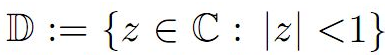of the form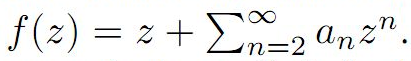For α≥0, the subclass B1α of S of Bazilevic functions has been extensively studied. In this paper we determine various properties of a subclass of B1α, for α≥0 which extends early results of a class of starlike functions studied by Ram Singh.

1: Paper Source PDF document

Paper's Title:

An Existence of the Solution to Neutral Stochastic Functional Differential Equations Under the Holder Condition

Author(s):

Young-Ho Kim

Department of Mathematics,
Changwon National University,
Changwon, Gyeongsangnam-do 51140,
Korea.

E-mail: iyhkim@changwon.ac.kr

Abstract:

In this paper, we show the existence and uniqueness of solution of the neutral stochastic functional differential equations under weakened H\"{o}lder condition, a weakened linear growth condition, and a contractive condition. Furthermore, in order to obtain the existence of a solution to the equation we used the Picard sequence.

1: Paper Source PDF document

Paper's Title:

Analysis of a Frictional Contact Problem for Viscoelastic Piezoelectric Materials

Author(s):

Meziane Said Ameur, Tedjani Hadj Ammar and Laid Maiza

Departement of Mathematics,
El Oued University,
P.O. Box 789, 39000 El Oued,
Algeria.
E-mail: said-ameur-meziane@univ-eloued.dz

Departement of Mathematics,
El Oued University,
P.O. Box 789, 39000 El Oued,
Algeria.

Department of Mathematics,
Kasdi Merbah University,
30000 Ouargla,
Algeria.
E-mail: maiza.laid@univ-ouargla.dz

Abstract:

In this paper, we consider a mathematical model that describes the quasi-static process of contact between two thermo-electro-viscoelastic bodies with damage and adhesion. The damage of the materials caused by elastic deformations. The contact is frictional and modeled with a normal compliance condition involving adhesion effect of contact surfaces. Evolution of the bonding field is described by a first order differential equation. We derive variational formulation for the model and prove an existence and uniqueness result of the weak solution. The proof is based on arguments of evolutionary variational inequalities, parabolic inequalities, differential equations, and fixed point theorem.

1: Paper Source PDF document

Paper's Title:

Riemann-Stieltjes Integrals and Some Ostrowski Type Inequalities

Author(s):

W. G. Alshanti

Department of General Studies,
Jubail University College,
KSA.
E-mail: shantiw@ucj.edu.sa

Abstract:

In this article, we investigate new integral inequalities of Ostrowski's type of various functional aspects. For mapping's second derivative, we assume two cases, namely, L1 and L spaces. Moreover, for first derivative, we investigate two different characteristics, namely, bounded variation and locally Lipchitz continuity. Applications to special means and composite quadrature rules are also carried out.

1: Paper Source PDF document

Paper's Title:

A Review on Minimally Supported Frequency Wavelets

Author(s):

K Pallavi1, M C Lineesh1, A Noufal2

1Department of Mathematics,
National Institute of Technology Calicut,
Kerala 673601,
India.
E-mail:
pavikrishnakumar@gmail.com
lineesh@nitc.ac.in

2Department of Mathematics,
Cochin University of Science and Technology,
Kerala 682022,
India.
E-mail: noufal@cusat.ac.in

Abstract:

This paper provides a review on Minimally Supported Frequency (MSF) wavelets that includes the construction and characterization of MSF wavelets. The characterization of MSF wavelets induced from an MRA is discussed and the nature of the low-pass filter associated with it is explained. The concept of wavelet set and dimension function is introduced to study this class of wavelets. Along with MSF wavelets, s-elementary wavelets and unimodular wavelets are also considered due to the similarity in definitions. Examples and illustrations are provided for more clarity.

Search and serve lasted 0 second(s).